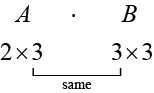# 7.5 Matrices and matrix operations  (Page 3/10)

 Page 3 / 10
Lab A Lab B
Computers 15 27
Computer Tables 16 34
Chairs 16 34

Converting the data to a matrix, we have

${C}_{2013}=\left[\begin{array}{c}15\\ 16\\ 16\end{array}\text{\hspace{0.17em}}\text{\hspace{0.17em}}\text{\hspace{0.17em}}\text{\hspace{0.17em}}\text{\hspace{0.17em}}\text{\hspace{0.17em}}\text{\hspace{0.17em}}\begin{array}{c}27\\ 34\\ 34\end{array}\right]$

To calculate how much computer equipment will be needed, we multiply all entries in matrix $\text{\hspace{0.17em}}C\text{\hspace{0.17em}}$ by 0.15.

$\left(0.15\right){C}_{2013}=\left[\begin{array}{c}\left(0.15\right)15\\ \left(0.15\right)16\\ \left(0.15\right)16\end{array}\text{\hspace{0.17em}}\text{\hspace{0.17em}}\text{\hspace{0.17em}}\text{\hspace{0.17em}}\text{\hspace{0.17em}}\text{\hspace{0.17em}}\text{\hspace{0.17em}}\text{\hspace{0.17em}}\begin{array}{c}\left(0.15\right)27\\ \left(0.15\right)34\\ \left(0.15\right)34\end{array}\right]=\left[\begin{array}{c}2.25\\ 2.4\\ 2.4\end{array}\text{\hspace{0.17em}}\text{\hspace{0.17em}}\text{\hspace{0.17em}}\text{\hspace{0.17em}}\text{\hspace{0.17em}}\begin{array}{c}4.05\\ 5.1\\ 5.1\end{array}\right]$

We must round up to the next integer, so the amount of new equipment needed is

$\left[\begin{array}{c}3\\ 3\\ 3\end{array}\text{\hspace{0.17em}}\text{\hspace{0.17em}}\text{\hspace{0.17em}}\text{\hspace{0.17em}}\text{\hspace{0.17em}}\begin{array}{c}5\\ 6\\ 6\end{array}\right]$

Adding the two matrices as shown below, we see the new inventory amounts.

$\left[\begin{array}{c}15\\ 16\\ 16\end{array}\text{\hspace{0.17em}}\text{\hspace{0.17em}}\text{\hspace{0.17em}}\text{\hspace{0.17em}}\text{\hspace{0.17em}}\text{\hspace{0.17em}}\text{\hspace{0.17em}}\begin{array}{c}27\\ 34\\ 34\end{array}\right]+\left[\begin{array}{c}3\\ 3\\ 3\end{array}\text{\hspace{0.17em}}\text{\hspace{0.17em}}\text{\hspace{0.17em}}\text{\hspace{0.17em}}\text{\hspace{0.17em}}\begin{array}{c}5\\ 6\\ 6\end{array}\right]=\left[\begin{array}{c}18\\ 19\\ 19\end{array}\text{\hspace{0.17em}}\text{\hspace{0.17em}}\text{\hspace{0.17em}}\text{\hspace{0.17em}}\text{\hspace{0.17em}}\begin{array}{c}32\\ 40\\ 40\end{array}\right]$

This means

${C}_{2014}=\left[\begin{array}{c}18\\ 19\\ 19\end{array}\text{\hspace{0.17em}}\text{\hspace{0.17em}}\text{\hspace{0.17em}}\text{\hspace{0.17em}}\text{\hspace{0.17em}}\begin{array}{c}32\\ 40\\ 40\end{array}\right]$

Thus, Lab A will have 18 computers, 19 computer tables, and 19 chairs; Lab B will have 32 computers, 40 computer tables, and 40 chairs.

## Scalar multiplication

Scalar multiplication involves finding the product of a constant by each entry in the matrix. Given

$A=\left[\begin{array}{cccc}{a}_{11}& & & {a}_{12}\\ {a}_{21}& & & {a}_{22}\end{array}\right]$

the scalar multiple $\text{\hspace{0.17em}}cA\text{\hspace{0.17em}}$ is

Scalar multiplication is distributive. For the matrices $\text{\hspace{0.17em}}A,B,$ and $\text{\hspace{0.17em}}C\text{\hspace{0.17em}}$ with scalars $\text{\hspace{0.17em}}a\text{\hspace{0.17em}}$ and $\text{\hspace{0.17em}}b,$

$\begin{array}{l}\\ \begin{array}{c}a\left(A+B\right)=aA+aB\\ \left(a+b\right)A=aA+bA\end{array}\end{array}$

## Multiplying the matrix by a scalar

Multiply matrix $\text{\hspace{0.17em}}A\text{\hspace{0.17em}}$ by the scalar 3.

$A=\left[\begin{array}{cc}8& 1\\ 5& 4\end{array}\right]$

Multiply each entry in $\text{\hspace{0.17em}}A\text{\hspace{0.17em}}$ by the scalar 3.

Given matrix $\text{\hspace{0.17em}}B,\text{}$ find $\text{\hspace{0.17em}}-2B\text{\hspace{0.17em}}$ where

$B=\left[\begin{array}{cc}4& 1\\ 3& 2\end{array}\right]$

$-2B=\left[\begin{array}{cc}-8& -2\\ -6& -4\end{array}\right]$

## Finding the sum of scalar multiples

Find the sum $\text{\hspace{0.17em}}3A+2B.$

First, find $\text{\hspace{0.17em}}3A,\text{}$ then $\text{\hspace{0.17em}}2B.$

$\begin{array}{l}\begin{array}{l}\hfill \\ \hfill \\ 3A=\left[\begin{array}{lll}3\cdot 1\hfill & \text{\hspace{0.17em}}\text{\hspace{0.17em}}3\left(-2\right)\hfill & \text{\hspace{0.17em}}\text{\hspace{0.17em}}3\cdot 0\hfill \\ 3\cdot 0\hfill & \text{\hspace{0.17em}}\text{\hspace{0.17em}}3\left(-1\right)\hfill & \text{\hspace{0.17em}}\text{\hspace{0.17em}}3\cdot 2\hfill \\ 3\cdot 4\hfill & \text{\hspace{0.17em}}\text{\hspace{0.17em}}3\cdot 3\hfill & \text{\hspace{0.17em}}\text{\hspace{0.17em}}3\left(-6\right)\hfill \end{array}\right]\hfill \end{array}\hfill \\ \text{\hspace{0.17em}}\text{\hspace{0.17em}}\text{\hspace{0.17em}}\text{\hspace{0.17em}}\text{\hspace{0.17em}}\text{\hspace{0.17em}}\text{\hspace{0.17em}}=\left[\begin{array}{rrr}\hfill 3& \hfill \text{\hspace{0.17em}}\text{\hspace{0.17em}}-6& \hfill \text{\hspace{0.17em}}\text{\hspace{0.17em}}0\\ \hfill 0& \hfill \text{\hspace{0.17em}}\text{\hspace{0.17em}}-3& \hfill \text{\hspace{0.17em}}\text{\hspace{0.17em}}6\\ \hfill 12& \hfill \text{\hspace{0.17em}}\text{\hspace{0.17em}}9& \hfill \text{\hspace{0.17em}}\text{\hspace{0.17em}}-18\end{array}\right]\hfill \end{array}$
$\begin{array}{l}\begin{array}{l}\hfill \\ \hfill \\ 2B=\left[\begin{array}{lll}2\left(-1\right)\hfill & \text{\hspace{0.17em}}\text{\hspace{0.17em}}2\cdot 2\hfill & \text{\hspace{0.17em}}\text{\hspace{0.17em}}2\cdot 1\hfill \\ 2\cdot 0\hfill & \text{\hspace{0.17em}}\text{\hspace{0.17em}}2\left(-3\right)\hfill & \text{\hspace{0.17em}}\text{\hspace{0.17em}}2\cdot 2\hfill \\ 2\cdot 0\hfill & \text{\hspace{0.17em}}\text{\hspace{0.17em}}2\cdot 1\hfill & \text{\hspace{0.17em}}\text{\hspace{0.17em}}2\left(-4\right)\hfill \end{array}\right]\hfill \end{array}\hfill \\ \text{\hspace{0.17em}}\text{\hspace{0.17em}}\text{\hspace{0.17em}}\text{\hspace{0.17em}}\text{\hspace{0.17em}}\text{\hspace{0.17em}}\text{\hspace{0.17em}}=\left[\begin{array}{rrr}\hfill -2& \hfill 4& \hfill 2\\ \hfill 0& \hfill -6& \hfill 4\\ \hfill 0& \hfill 2& \hfill -8\end{array}\right]\hfill \end{array}$

Now, add $\text{\hspace{0.17em}}3A+2B.$

## Finding the product of two matrices

In addition to multiplying a matrix by a scalar, we can multiply two matrices. Finding the product of two matrices is only possible when the inner dimensions are the same, meaning that the number of columns of the first matrix is equal to the number of rows of the second matrix. If $\text{\hspace{0.17em}}A\text{\hspace{0.17em}}$ is an matrix and $\text{\hspace{0.17em}}B\text{\hspace{0.17em}}$ is an matrix, then the product matrix $\text{\hspace{0.17em}}AB\text{\hspace{0.17em}}$ is an matrix. For example, the product $\text{\hspace{0.17em}}AB\text{\hspace{0.17em}}$ is possible because the number of columns in $\text{\hspace{0.17em}}A\text{\hspace{0.17em}}$ is the same as the number of rows in $\text{\hspace{0.17em}}B.\text{\hspace{0.17em}}$ If the inner dimensions do not match, the product is not defined.We multiply entries of $\text{\hspace{0.17em}}A\text{\hspace{0.17em}}$ with entries of $\text{\hspace{0.17em}}B\text{\hspace{0.17em}}$ according to a specific pattern as outlined below. The process of matrix multiplication becomes clearer when working a problem with real numbers.

To obtain the entries in row $\text{\hspace{0.17em}}i\text{\hspace{0.17em}}$ of $\text{\hspace{0.17em}}AB,\text{}$ we multiply the entries in row $\text{\hspace{0.17em}}i\text{\hspace{0.17em}}$ of $\text{\hspace{0.17em}}A\text{\hspace{0.17em}}$ by column $\text{\hspace{0.17em}}j\text{\hspace{0.17em}}$ in $\text{\hspace{0.17em}}B\text{\hspace{0.17em}}$ and add. For example, given matrices $\text{\hspace{0.17em}}A\text{\hspace{0.17em}}$ and $\text{\hspace{0.17em}}B,\text{}$ where the dimensions of $\text{\hspace{0.17em}}A\text{\hspace{0.17em}}$ are and the dimensions of $\text{\hspace{0.17em}}B\text{\hspace{0.17em}}$ are the product of $\text{\hspace{0.17em}}AB\text{\hspace{0.17em}}$ will be a matrix.

Multiply and add as follows to obtain the first entry of the product matrix $\text{\hspace{0.17em}}AB.$

1. To obtain the entry in row 1, column 1 of $\text{\hspace{0.17em}}AB,\text{}$ multiply the first row in $\text{\hspace{0.17em}}A\text{\hspace{0.17em}}$ by the first column in $\text{\hspace{0.17em}}B,$ and add.
$\left[\begin{array}{ccc}{a}_{11}& {a}_{12}& {a}_{13}\end{array}\right]\cdot \left[\begin{array}{c}{b}_{11}\\ {b}_{21}\\ {b}_{31}\end{array}\right]={a}_{11}\cdot {b}_{11}+{a}_{12}\cdot {b}_{21}+{a}_{13}\cdot {b}_{31}$
2. To obtain the entry in row 1, column 2 of $\text{\hspace{0.17em}}AB,\text{}$ multiply the first row of $\text{\hspace{0.17em}}A\text{\hspace{0.17em}}$ by the second column in $\text{\hspace{0.17em}}B,$ and add.
$\left[\begin{array}{ccc}{a}_{11}& {a}_{12}& {a}_{13}\end{array}\right]\cdot \left[\begin{array}{c}{b}_{12}\\ {b}_{22}\\ {b}_{32}\end{array}\right]={a}_{11}\cdot {b}_{12}+{a}_{12}\cdot {b}_{22}+{a}_{13}\cdot {b}_{32}$
3. To obtain the entry in row 1, column 3 of $\text{\hspace{0.17em}}AB,\text{}$ multiply the first row of $\text{\hspace{0.17em}}A\text{\hspace{0.17em}}$ by the third column in $\text{\hspace{0.17em}}B,$ and add.
$\left[\begin{array}{ccc}{a}_{11}& {a}_{12}& {a}_{13}\end{array}\right]\cdot \left[\begin{array}{c}{b}_{13}\\ {b}_{23}\\ {b}_{33}\end{array}\right]={a}_{11}\cdot {b}_{13}+{a}_{12}\cdot {b}_{23}+{a}_{13}\cdot {b}_{33}$

how do I set up the problem?
what is a solution set?
Harshika
find the subring of gaussian integers?
Rofiqul
hello, I am happy to help!
Abdullahi
find the value of 2x=32
divide by 2 on each side of the equal sign to solve for x
corri
X=16
Michael
Want to review on complex number 1.What are complex number 2.How to solve complex number problems.
Beyan
use the y -intercept and slope to sketch the graph of the equation y=6x
how do we prove the quadratic formular
hello, if you have a question about Algebra 2. I may be able to help. I am an Algebra 2 Teacher
thank you help me with how to prove the quadratic equation
Seidu
may God blessed u for that. Please I want u to help me in sets.
Opoku
what is math number
4
Trista
x-2y+3z=-3 2x-y+z=7 -x+3y-z=6
Need help solving this problem (2/7)^-2
x+2y-z=7
Sidiki
what is the coefficient of -4×
-1
Shedrak
the operation * is x * y =x + y/ 1+(x × y) show if the operation is commutative if x × y is not equal to -1
An investment account was opened with an initial deposit of \$9,600 and earns 7.4% interest, compounded continuously. How much will the account be worth after 15 years?
lim x to infinity e^1-e^-1/log(1+x)
given eccentricity and a point find the equiationByByBy Subramanian DivyaBy Stephen VoronBy Briana HamiltonByBy OpenStaxBy Keyaira BraxtonBy OpenStaxBy OpenStaxBy Brooke DelaneyBy Wey Hey